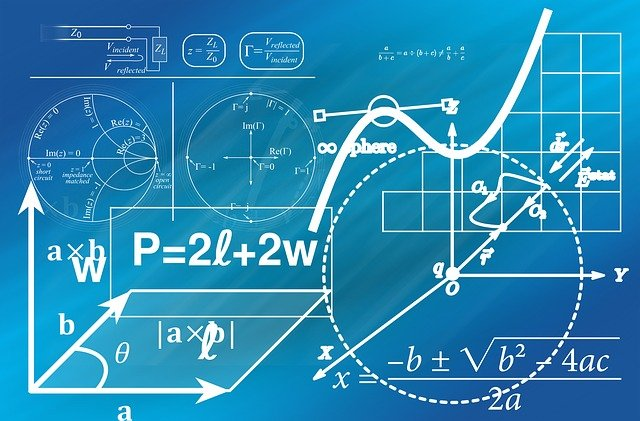## Physics for XIIPhysics is one of the most fundamental discipline of science its main goal is to understand how the universe behave. If you have so many questions regarding the universe like- why sky is blue? How rainbow is formed? Can light bend around corners? Does an atom have a color? and many more. You can get your all answers in this course. This course is designed in such a way; it will enhance your theoretical understanding as well as problem solving skills. So you can write your 12th exam and same time you can try to qualify a competitive exam like IIT-JEE or NEET.

## course syllabus• 1.1 Introduction
• 1.2 Electric Charge
• 1.3 Conductors and Insulators
• 1.4 Charging by Induction
• 1.5 Basic Properties of Electric Charge
• 1.6 Coulomb’s Law
• 1.7 Forces between Multiple Charges
• 1.8 Electric Field
• 1.9 Electric Field Lines
• 1.10 Electric Flux
• 1.11 Electric Dipole
• 1.12 Dipole in a Uniform External Field
• 1.13 Continuous Charge Distribution
• 1.14 Gauss’s Law
• 1.15 Applications of Gauss’s Law
• 2.1 Introduction
• 2.2 Electrostatic Potential
• 2.3 Potential due to a Point Charge
• 2.4 Potential due to an Electric Dipole
• 2.5 Potential due to a System of Charges
• 2.6 Equipotential Surfaces
• 2.7 Potential Energy of a System of Charges
• 2.8 Potential Energy in an External Field
• 2.9 Electrostatics of Conductors
• 2.10 Dielectrics and Polarisation
• 2.11 Capacitors and Capacitance
• 2.12 The Parallel Plate Capacitor
• 2.13 Effect of Dielectric on Capacitance
• 2.14 Combination of Capacitors
• 2.15 Energy Stored in a Capacitor
• 2.16 Van de Graaff Generator
• 3.1 Introduction
• 3.2 Electric Current
• 3.3 Electric Currents in Conductors
• 3.4 Ohm’s law
• 3.5 Drift of Electrons and the Origin of Resistivity
• 3.6 Limitations of Ohm’s Law
• 3.7 Resistivity of Various Materials
• 3.8 Temperature Dependence of Resistivity
• 3.9 Electrical Energy, Power
• 3.10 Combination of Resistors — Series and Parallel
• 3.11 Cells, emf, Internal Resistance
• 3.12 Cells in Series and in Parallel
• 3.13 Kirchhoff’s Rules
• 3.14 Wheatstone Bridge
• 3.15 Meter Bridge
• 3.16 Potentiometer
• 4.1 Introduction
• 4.2 Magnetic Force
• 4.3 Motion in a Magnetic Field
• 4.4 Motion in Combined Electric and Magnetic Fields
• 4.5 Magnetic Field due to a Current Element, Biot-Savart Law
• 4.6 Magnetic Field on the Axis of a Circular Current Loop
• 4.7 Ampere’s Circuital Law
• 4.8 The Solenoid and the Toroid
• 4.9 Force between Two Parallel Currents, the Ampere
• 4.10 Torque on Current Loop, Magnetic Dipole
• 4.11 The Moving Coil Galvanometer
• 5.1 Introduction
• 5.2 The Bar Magnet
• 5.3 Magnetism and Gauss’s Law
• 5.4 The Earth’s Magnetism
• 5.5 Magnetisation and Magnetic Intensity
• 5.6 Magnetic Properties of Materials
• 5.7 Permanent Magnets and Electromagnets
• 6.1 Introduction
• 6.2 The Experiments of Faraday and Henry
• 6.3 Magnetic Flux
• 6.4 Faraday’s Law of Induction
• 6.5 Lenz’s Law and Conservation of Energy
• 6.6 Motional Electromotive Force
• 6.7 Energy Consideration: A Quantitative Study
• 6.8 Eddy Currents
• 6.9 Inductance
• 6.10 AC Generator
• 7.1 Introduction
• 7.2 AC Voltage Applied to a Resistor
• 7.3 Representation of AC Current and Voltage by Rotating Vectors — Phasors
• 7.4 AC Voltage Applied to an Inductor
• 7.5 AC Voltage Applied to a Capacitor
• 7.6 AC Voltage Applied to a Series LCR Circuit
• 7.7 Power in AC Circuit: The Power Factor
• 7.8 LC Oscillations
• 7.9 Transformers
• 8.1 Introduction
• 8.2 Displacement Current
• 8.3 Electromagnetic Waves
• 8.4 Electromagnetic Spectrum
• 9.1 Introduction
• 9.2 Reflection of Light by Spherical Mirrors
• 9.3 Refraction
• 9.4 Total Internal Reflection
• 9.5 Refraction at Spherical Surfaces and by Lenses
• 9.6 Refraction through a Prism
• 9.7 Dispersion by a Prism
• 9.8 Some Natural Phenomena due to Sunlight
• 9.9 Optical Instruments
• 10.1 Introduction
• 10.2 Huygens Principle
• 10.3 Refraction and Reflection of Plane Waves using Huygens Principle
• 10.4 Coherent and Incoherent Addition of Waves
• 10.5 Interference of Light Waves and Young’s Experiment
• 10.6 Diffraction
• 10.7 Polarisation
• 11.1 Introduction
• 11.2 Electron Emission
• 11.3 Photoelectric Effect
• 11.4 Experimental Study of Photoelectric Effect
• 11.5 Photoelectric Effect and Wave Theory of Light
• 11.6 Einstein’s Photoelectric Equation: Energy Quantum of Radiation
• 11.7 Particle Nature of Light: The Photon
• 11.8 Wave Nature of Matter
• 11.9 Davisson and Germer Experiment
• 12.1 Introduction
• 12.2 Alpha-particle Scattering and Rutherford’s Nuclear Model of Atom
• 12.3 Atomic Spectra
• 12.4 Bohr Model of the Hydrogen Atom
• 12.5 The Line Spectra of the Hydrogen Atom
• 12.6 DE Broglie’s Explanation of Bohr’s Second Postulate of Quantisation
• 13.1 Introduction
• 13.2 Atomic Masses and Composition of Nucleus
• 13.3 Size of the Nucleus
• 13.4 Mass-Energy and Nuclear Binding Energy
• 13.5 Nuclear Force
• 13.7 Nuclear Energy
• 14.1 Introduction
• 14.2 Classification of Metals, Conductors and Semiconductors
• 14.3 Intrinsic Semiconductor
• 14.4 Extrinsic Semiconductor
• 14.5 p-n Junction
• 14.6 Semiconductor Diode
• 14.7 Application of Junction Diode as a Rectifier
• 14.8 Special Purpose p-n Junction Diodes
• 14.9 Junction Transistor
• 14.10 Digital Electronics and Logic Gates
• 14.11 Integrated Circuits
• 15.1 Introduction
• 15.2 Elements of a Communication System
• 15.3 Basic Terminology Used in Electronic Communication Systems
• 15.4 Bandwidth of Signals
• 15.5 Bandwidth of Transmission Medium
• 15.6 Propagation of Electromagnetic Waves
• 15.7 Modulation and its Necessity
• 15.8 Amplitude Modulation
• 15.9 Production of Amplitude Modulated Wave
• 15.10 Detection of Amplitude Modulated Wave